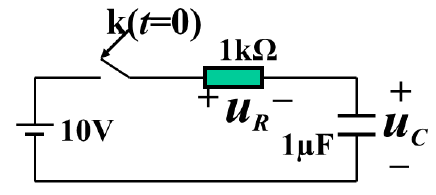【简答题】Fill in the blankFor the circuit, if we list the ODE with the voltageuRas the variable, we can getduRdt+AuR=B; if we list the ODE with the voltageuCas the variable, we can getduCdt+CuC=D. In these ODEs, A=__and C=__A.

B.

C.

D.

A.

B.

C.

D.

A.

B.

C.

D.

A.

B.

C.

D.

A.
1
B.
2
C.
3
D.
4

A.

B.

C.

D.

A.

B.

C.

D.

A.

B.

C.

D.

A.

B.

C.

D.

A.

B.

C.

D.

A.

B.

C.

D.

A.

B.

C.

D.

A.
B.
C.
D.

A.

B.

C.

D.

KYT活动原则中预防是指将（ ）放在各项工作的首位。
A.

B.

C.

D.

A.
55
B.
60
C.
70
D.
75

A.

B.

C.

D.

A.

B.

C.

D.

A.
60
B.
120
C.
100
D.
80

A.

B.

C.

D.

A.

B.

C.

D.

A.

B.

C.

D.

A.

B.

C.

D.

A.
1
B.
6
C.
5
D.
8

A.

B.

C.

D.

A.

B.

C.

D.

A.

B.

C.

D.

A.

B.

C.

D.

A.

B.

C.

D.

A.

B.

C.

D.

A.

B.

C.

D.

A.

B.

C.

D.

A.

B.

C.

D.

A.
B.
C.

D.

E.

A.

B.

C.

D.

E.

KYT的推进方法分为（ ）。
A.

B.

C.

D.

E.

A.

B.

C.

D.

E.

A.

B.

C.

D.

E.

A.

B.

C.

D.

E.

A.

B.

C.

D.

E.

A.

B.

C.

D.

E.

A.

B.

C.

D.

E.

A.

B.

C.

D.

E.

A.

B.

C.

D.

E.

A.

B.

C.

D.

E.

A.

B.

C.

D.

E.

A.

B.

A.

B.

A.

B.

A.

B.

“吓一跳、冒冷汗”活动中，现场主管在确认内容时，不需要对事件等级进行划分。（ ）
A.

B.

A.

B.

A.

B.

A.

B.

A.

B.

A.

B.

A.

B.

A.

B.

A.

B.

A.

B.

A.

B.

A.

B.

A.

B.

A.

B.

KYT开展的目的是控制作业过程中的危险，分析和制止可能出现的事故。（ ）
A.

B.

KYT活动原则中预防是指将预防放在各项工作的首位，将技术措施置于预防的优先。（ ）
A.

B.

KYT危险预知训练是讨论分析作业过程中潜在的危险因素，并避免事故发生。（ ）
A.

B.

A.

B.

A.

B.

KYT的作用是深入讨论分析危险因素、提高对危险的感受性。（ ）
A.

B.

A.

B.

A.

B.

A.

B.

A.

B.

A.

B.

A.

B.

A.

B.

A.

B.

A.

B.

A.
8
B.
9
C.
10
D.
12

A.

B.

C.

D.

A.

B.

C.

D.

WS180×2000CNCd型数控轧辊磨床的最大磨削长度是2m。（ ）
A.

B.

（ ）是造成外圆磨削表面粗糙度值大的主要原因。
A.

B.

C.

D.

A.

B.

C.

D.

A.

B.

C.

D.

A.

B.

C.

D.

E.

A.

B.

C.

D.

E.

WS450×5500CNCd型数控轧辊磨床的最大磨削长度是5.5m。（ ）
A.

B.

A.

B.

C.

D.

E.

A.

B.

C.

D.

A.

B.

C.

D.

E.

WS450×5500CNCd型数控轧辊磨床的最大磨削直径是900mm。（ ）
A.

B.

A.

B.

A.

B.

C.

D.

E.

A.

B.

C.

D.

E.

A.

B.

A.

B.

A.

B.

A.

B.打开"刷刷题APP"，使用更方便